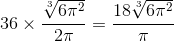# ISEE Upper Level Quantitative : How to find the radius of a sphere

## Example Questions

### Example Question #1 : Spheres

The volume of a sphere is one cubic yard. Give its radius in inches.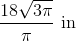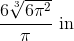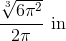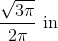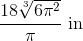Explanation:

The volumeof a sphere with radiusis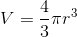.

To find the radius in yards, we set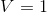and solve for.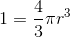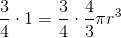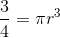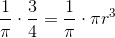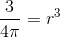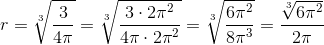yards.

Since the problem requests the radius in inches, multiply by 36: